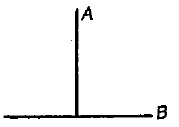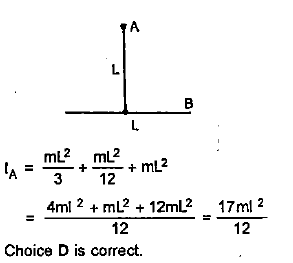A T joint is formed by two identical rods A and B each of mass m and length L in the XY plane as shown. Its moment of inertia about axis passing through A and perpendicular to the plane of the joint,1.  $\frac{2{\mathrm{mL}}^{2}}{3}$

2.  $\frac{{\mathrm{mL}}^{2}}{12}$

3.  $\frac{{\mathrm{mL}}^{2}}{6}$

4.  None of these

Concept Videos :-

#10 | Moment of Inertia (MI): Introduction
#11 | MI: Discreet Particles
#12 | MI: Continuous Bodies_1
#13 | MI: Questions on Continuous Bodies
#14 | MI: Continuous Bodies_2
#15 | Theorems of Moment of Inertia
#16 | Questions on Theorems of MI
#17 | Set 1: Previous Year Problems

Concept Questions :-

Moment of inertiaDifficulty Level:

• 17%
• 37%
• 15%
• 33%